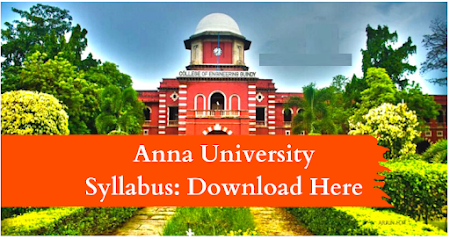### MA8352 LINEAR ALGEBRA AND PARTIAL DIFFERENTIAL EQUATIONS L T P C 4 0 0 4

OBJECTIVES:
 To introduce the basic notions of groups, rings, fields which will then be used to solve related problems.  To understand the concepts of vector space, linear transformations and diagonalization.  To apply the concept of inner product spaces in orthogonalization.  To understand the procedure to solve partial differential equations.  To give an integrated approach to number theory and abstract algebra, and provide a firm basis for further reading and study in the subject.

UNIT I    VECTOR SPACES                                                                                                            12 Vector spaces – Subspaces – Linear combinations and linear system of equations – Linear independence and linear dependence – Bases and dimensions.

UNIT II   LINEAR TRANSFORMATION AND DIAGONALIZATION                                   12 Linear transformation - Null spaces and ranges - Dimension theorem - Matrix representation of a linear transformations - Eigenvalues and eigenvectors - Diagonalizability.

UNIT III  INNER PRODUCT SPACES                                                                                          12 Inner product, norms - Gram Schmidt orthogonalization process - Adjoint of linear operations - Least square approximation.

UNIT IV  PARTIAL DIFFERENTIAL EQUATIONS                                                                   12 Formation – Solutions of first order equations – Standard types and equations reducible to standard types – Singular solutions – Lagrange‘s linear equation – Integral surface passing through a given curve – Classification of partial differential equations - Solution of linear equations of higher order with constant coefficients – Linear non-homogeneous partial differential equations.

UNIT V   FOURIER SERIES SOLUTIONS OF PARTIAL DIFFERENTIAL EQUATIONS   12 Dirichlet‘s conditions – General Fourier series – Half range sine and cosine series - Method of separation of variables – Solutions of one dimensional wave equation and one-dimensional heat equation – Steady state solution of two-dimensional heat equation – Fourier series solutions in Cartesian coordinates.

TOTAL: 60 PERIODS
OUTCOMES: Upon successful completion of the course, students should be able to:  Explain the fundamental concepts of advanced algebra and their role in modern mathematics and applied contexts.  Demonstrate accurate and efficient use of advanced algebraic techniques.  Demonstrate their mastery by solving non - trivial problems related to the concepts and by proving simple theorems about the statements proven by the text.  Able to solve various types of partial differential equations. Able to solve engineering problems using Fourier series.

TEXTBOOKS:
1. Grewal B.S., ―Higher Engineering Mathematics‖, Khanna Publishers, New Delhi, 43rd Edition, 2014.
2. Friedberg, A.H., Insel, A.J. and Spence, L., ―Linear Algebra‖, Prentice Hall of India, New Delhi, 2004.

REFERENCES:
1. Burden, R.L. and Faires, J.D, "Numerical Analysis", 9th Edition, Cengage Learning, 2016.
2. James, G. ―Advanced Modern Engineering Mathematics‖, Pearson Education, 2007.
3. Kolman, B. Hill, D.R., ―Introductory Linear Algebra‖, Pearson Education, New Delhi, First Reprint, 2009.
4. Kumaresan, S., ―Linear Algebra – A Geometric Approach‖, Prentice – Hall of India, New Delhi, Reprint, 2010.
5. Lay, D.C., ―Linear Algebra and its Applications‖, 5th Edition, Pearson Education, 2015.
6. O‘Neil, P.V., ―Advanced Engineering Mathematics‖, Cengage Learning, 2007.
7. Strang, G., ―Linear Algebra and its applications‖, Thomson (Brooks/Cole), New Delhi, 2005.
8. Sundarapandian, V. ―Numerical Linear Algebra‖, Prentice Hall of India, New Delhi, 2008.